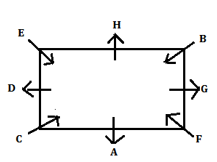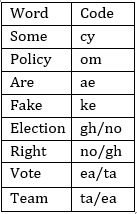Latest Banking jobs   »   Reasoning Ability Quiz For IBPS Clerk/NIACL...

# Reasoning Ability Quiz For IBPS Clerk/NIACL AO Prelims 2021- 30th August

Directions (1-5): Study the information carefully and answer the questions given below.
Eight persons i.e. A, B, C, D, E, F, G and H sit at square table. Four of them sit at corners and face inside. Remaining four sits at middle of the side and face outside. D sits second to the right of A. Two persons sit between D and F. G does not face D. H sits third to the right of F. B sits opposite to C. E sits second to the right of B.

Q1. Who among the following person sits second to the right of G?
(a) H
(b) A
(c) C
(d) E
(e) G

Q2. Who among the following person sits third to the right of C?
(a) G
(b) A
(c) F
(d) D
(e) H

Q3. How many persons sit between A and D when counted from left of A?
(a) One
(b) Two
(c) Three
(d) Five
(e) Four

Q4. Which of the following statement is true regarding E?
(a) E sits immediate right of B
(b) E sits second to the left of B
(c) None is true
(d) E sits at one of the corner
(e) E face outside

Q5. Four of the following five are alike in certain way based from a group, find the one that does not belong to that group?
(a) A-F
(b) B-H
(c) C-A
(d) D-E
(e) G-A

Directions (6-10): Study the information carefully and answer the questions given below.
In the coded language,
“Some Policy Are Fake” is coded as “om cy ke ae”
“Election Are Right Policy” is coded as “gh ae no om”
“Vote Some Are Team” is coded as “ea cy ae ta”

Q6. What is the code for the word “Are”?
(a) cy
(b) ke
(c) ae
(d) ta
(e) om

Q7. What is the code for the word “Right”?
(a) gh
(b) ta
(c) ae
(d) no
(e) Can’t be determined

Q8. What is the word for the code “ta”?
(a) Some
(b) Right
(c) Team
(d) Vote
(e) Either (c) or (d)

Q9. Which of the following options may be the code of “Election Are Policy” as per the above code?
(a) no ke om
(b) no ae gh
(c) gh ae no
(d) gh ae om
(e) gh ke no

Q10. What is the word for the code “om”?
(a) Policy
(b) Right
(c) Vote
(d) Election
(e) None of these

Q11. Rohit ranked 15th from the top and 23nd from the bottom in a class. How many students are there in the class?
(a) 38
(b) 39
(c) 37
(d) 36
(e) 40

Q12. Deepak ranked 31st from the bottom and 13th from the top among those who passed an exam. six boys did not participate in the exam and four failed in it. How many boys were there in the class?
(a) 54
(b) 55
(c) 52
(d) 53
(e) 51

Q13. In a row of boys, Aman is tenth from the right end and Babloo is eighth from the left end. If in this row Babloo is twelveth from the right then what is the position of Aman from the left?
(a) 9
(b) 10
(c) 11
(d) 12
(e) None of these

Q14. Harmaini is 19th from the left end of a row of 37 students and Harry is 16th from the right end in the same row. How many students are there between them in the row?
(a) 1
(b) 3
(c) 5
(d) 4
(e) 2

Q15. Sumit is 18th from the left end of a row and Adarsh is 10th from the right end of row. If they interchanged their positions then adarsh ranks become 22 from right end. Find total number of persons in the row?
(a) 40
(b) 39
(c) 38
(d) 41
(e) None of these

Solutions:

Solutions (1-5):
Sol.S1. Ans(b)
S2. Ans(a)
S3. Ans(d)
S4. Ans(d)
S5. Ans(e)

Solutions (6-10):
Sol.S6. Ans(c)
S7. Ans(e)
S8. Ans(e)
S9. Ans(d)
S10. Ans(a)

S11. Ans(c)
Sol. Number of students in the class= 15+23-1=37

S12. Ans(d)
Sol. Number of boys who passed = (31 + 13 – 1) = 43
∴ Total number of boys in the class = (43 + 6 + 4) = 53

S13. Ans(b)
Sol. Clearly, Aman is 10th from the right end and
Babloo is 8th from the left end and 12th from the right end of the row
So, number of boys in the row = (8 -1 + 12) = 19
Now, Aman is 10th from the right
Aman from left end= 19- (10-1)
Hence, Aman is 10th from the left end of the row.

S14. Ans(e)
Sol. Harmaini position from right end =(37+1-19)=19
Students between them=(19-16-1)=2

S15. Ans(b)
Sol.Total number of persons in the row=(18+22-1)=39